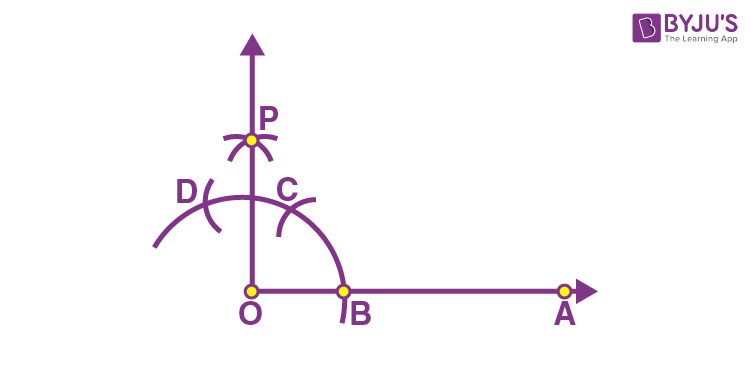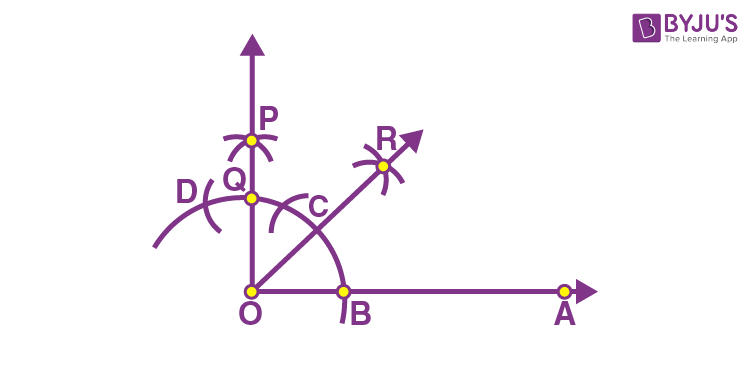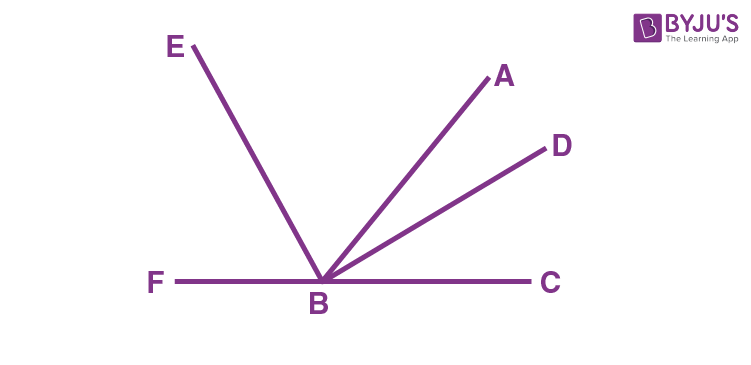# Class 9 Maths Chapter 11 Constructions MCQs

Class 9 Maths Chapter 11 Constructions MCQs are provided online here, with solved answers. The chapter-wise questions are prepared, as per the CBSE syllabus and NCERT curriculum. The objective questions are provided with detailed explanations. Also, check Important Questions for Class 9 Maths.

## MCQs on Class 9 Maths Chapter 11 Constructions

Solve the MCQs for constructions with each question provided with four options and select the right option.

1) Which of the following angles can be constructed using a ruler and compass?

a. 35°

b. 40°

c. 90°

d. 50°

Explanation: See the figure below:∠POA = 90°

2) Which of the following angles can be constructed using a ruler and compasses?

a. 35°

b. 45°

c. 95°

d. 55°

Explanation: See the figure below:∠ROA = 45°

3) Which of these angles we cannot construct it using a ruler and compasses?

a. 120°

b. 70°

c. 60°

d. All can be constructed

4) Which of these angles cannot be constructed using a ruler and compasses?

a. 120°

b. 60°

c. 140°

d. 135°

5) If a, b and c are the lengths of three sides of a triangle, then:

a. a+b>c

b. a-b>c

c. a+b=c

d. a-b=c

Explanation: The sum of two sides of a triangle is always greater than the third side.

6) Which of the following set of lengths can be the sides of a triangle?

a. 2 cm, 4 cm, 1.9 cm

b. 1.6 cm, 3.7 cm. 5.3 cm

c. 5.5 cm, 6.5 cm, 8.9 cm

d. None of the above

7) The side lengths 4cm, 4cm and 4cm can be sides of:

a. Scalene Triangle

b. Isosceles Triangle

c. Equilateral Triangle

d. None of the above

Explanation: Equilateral triangle has all its three sides equal.

8) To construct a bisector of a given angle, we need:

a. A ruler

b. A compass

c. A protractor

d. Both ruler and compass

9) To construct an angle of 60 degrees, we need to draw first:

a. A ray

b. An arc

c. Two rays

d. A straight line

Explanation: To construct an angle of 60 degrees we need to start at the initial point of a ray.

10) If we want to construct a triangle, given its perimeter, then we need to know:

a. Sum of two sides of triangle

b. Difference between two sides of triangle

c. One base angles

d. Two base angles

11) With the help of a ruler and a compass, it is possible to construct an angle of

(a) 35°

(b) 37.5°

(c) 40°

(d) 47.5°

Explanation: With the help of a ruler and compass, it is possible to construct an angle of 37.5°.

12) With the help of a ruler and a compass it is not possible to construct an angle of

(a) 22.5°

(b) 37.5°

(c) 40°

(d) 67.5°

Explanation: With the help of a ruler and a compass it is possible to construct the angles of 22.5°, 30°, 45°, 60° etc. (i.e)multiples of 15° and its angle bisector. Hence, it is not possible to construct a 40° angle using a ruler and compass.

13) The construction of a triangle ABC in which AB = 4 cm, ∠A = 60° is not possible when the difference of BC and AC is equal to

(a) 2.5 cm

(b) 3 cm

(c) 3.5 cm

(d) 4.5 cm

Explanation: Among the options given, 4.5 >4, since the difference of any two sides of a triangle cannot be greater than the third side.

14) A triangle ABC with AB = 4 cm and ∠A= 60° and ∠B= 40° is constructed. Then what is the measurement of ∠C?

(a) 40°

(b) 60°

(c) 80°

(d) 100°

Explanation:

By using the angle sum property of a triangle,

∠A+∠B+∠C = 180°

60°+40°+∠C = 180°

∠C = 180°-100°= 80°.

15) The construction of a triangle ABC, given that BC = 6 cm, ∠B = 45° is not possible when the difference of AB and AC is equal to:

(a) 4 cm

(b) 5 cm

(c) 5.2 cm

(d) 6.9 cm

Explanation: Among the options given, 6.9>6, since the difference of any two sides of a triangle cannot be greater than the third side.

16) The side length of 2 cm, 3 cm, and 4 cm can be the sides of

(a) Scalene triangle

(b) Isosceles triangle

(c) Equilateral triangle

(d) None of the above

Explanation: The side length of 2 cm, 3 cm, and 4 cm can be the sides of a scalene triangle, as all the sides have different measurements.

17) The side length of 5 cm, 3 cm, and 5 cm can be the sides of

(a) Scalene triangle

(b) Isosceles triangle

(c) Equilateral triangle

(d) None of the above

Explanation: The side length of 5 cm, 3 cm, and 5 cm can be the sides of an isosceles triangle, as two sides are of the same length and the length of one side of the triangle varies.

18) The internal and external bisectors of an angle forms

(a) Acute angle

(b) Right angle

(c) Obtuse angle

(d) Straight angle

Explanation: The internal and external bisectors of an angle forms the right angle.

Now, consider the below figure,Here, BD is the internal angle bisector and BE is the external bisector.

Hence, the angle formed between the internal and external bisector of an angle is the right angle.

19) The bisector of an angle lies in its

(a) Interior

(b) Exterior

(c) Anywhere in the plane

(d) On the arms of the angle

Explanation:  The bisector of an angle lies in its interior.

20) The point of occurrence of three angle bisectors of a triangle is called

(a) Incentre

(b) Circumcentre

(c) Orthocentre

(d) Centroid

Explanation:

The angle bisectors are the lines that bisect the angles of a triangle. The point of occurrence of three angle bisectors of a triangle is called incentre.

Stay tuned with BYJU’S – The Learning App and download the app today to get more class-wise concepts.